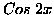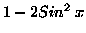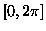Next: Symbolic Integration Up: The Problem Spaces Previous: Symbolic Regression

## Finding Trigonometric Identities

The second problem involves applying our system to a trigonometric identity problem summarised in Table 2 [Ryan 98b]. The particular function examined was, and the desired trigonometric identity was. The system was given a set of input and output pairs, and must determine the function that maps one onto the other, with the input values in the range. Other identities exist for, such as 2 Cos2x - 1. If we were to include Cos as one of the terminals in our BNF grammar definition, GE would naturally produce simple Cos identities for, which was verified in early experiments which included Cos. These runs consistently found the target expressionand, therefore, we decided to exclude Cos from the terminal operator set. Koza included the constant 1.0 in his terminal operator set, although Genetic Programming has shown an ability to generate the constant 1.0, as has GE with expressions such as x/x. However, we included 1.0 for reasons of consistency.

Table 2: Grammatical Evolution Tableau for finding Trigonometric Identities
 Objective : Find a new mathematical expression, in symbolic form that equals a given mathematical expression, for all values of its independent variables. Terminal Operands: X , the constant 1.0 Terminal Operators The binary operators +, *, /,- and the unary operator Sin Fitness cases The given sample of 20 data points in the interval [] Raw Fitness The sum, taken over the 20 fitness cases, of the error Standardised Fitness Same as raw fitness Hits The number of fitness cases for which the error is less than 0.01 Wrapper Standard productions to generate C functions Parameters M = 500, G = 51Next: Symbolic Integration Up: The Problem Spaces Previous: Symbolic Regression
root
1998-10-02